# Selina Solutions Concise Mathematics Class 6 Chapter 30 Revision Exercise Symmetry

Selina Solutions Concise Mathematics Class 6 Chapter 30 Revision Exercise Symmetry can be accessed by the students to understand the topics covered in an effective way. The solutions are curated by the subject matter experts in order to help students prepare for their exams effortlessly. All the solutions are in accordance with the latest ICSE guidelines, to help students score more marks. Continuous practice of these solutions helps them to obtain problem solving skills in Mathematics. For more in-depth knowledge about the concepts, students can avail Selina Solutions Concise Mathematics Class 6 Chapter 30 Revision Exercise Symmetry PDF, from the links which are given below.

Chapter 30 has problems on the concept of Symmetry. Regular revision of exercise problems helps students strengthen their knowledge pertaining to the chapter, which is important from an exam perspective. Solutions can be referred to cross check their answers and understand various ways of solving problems with ease.

## Selina Solutions Concise Mathematics Class 6 Chapter 30 Revision Exercise Symmetry Download PDF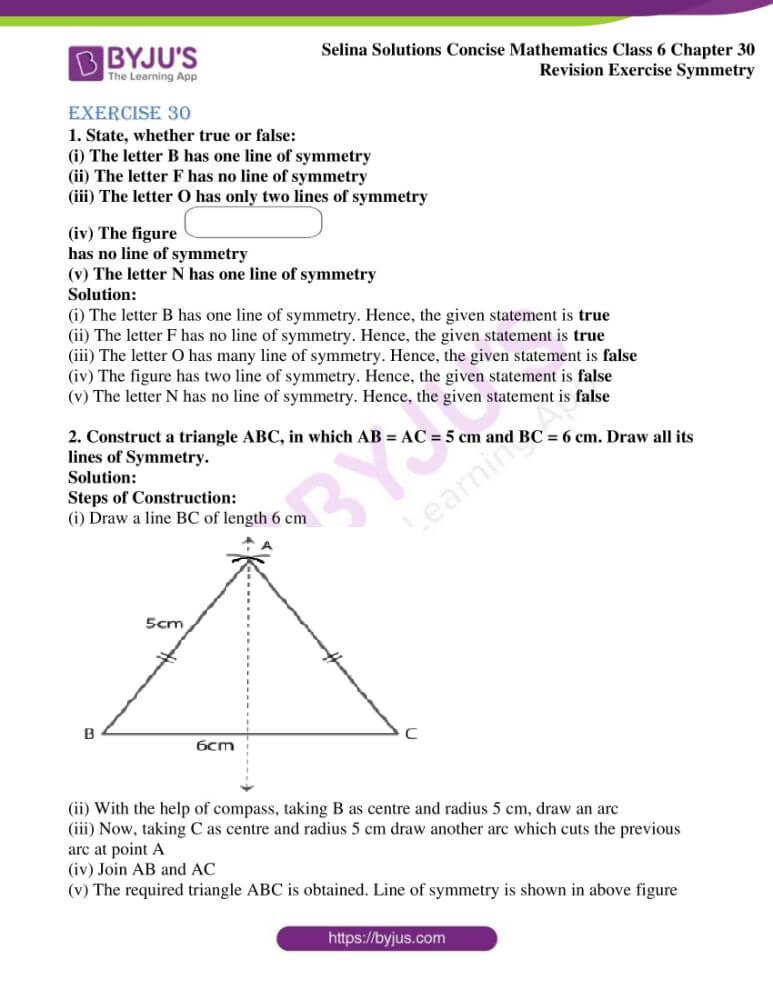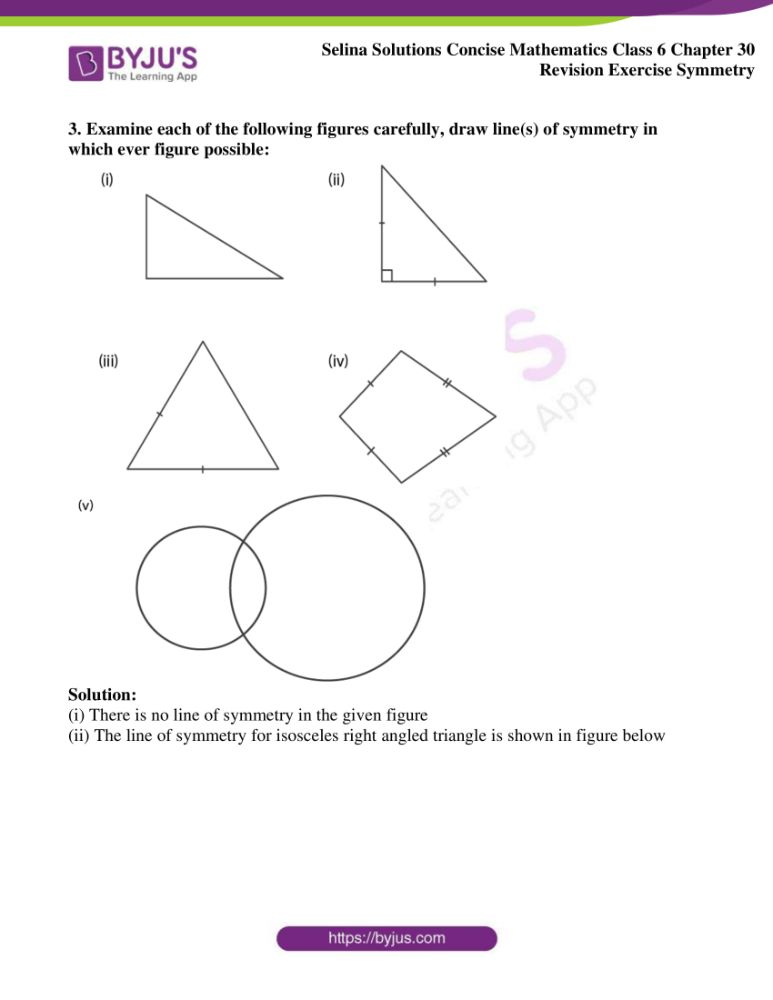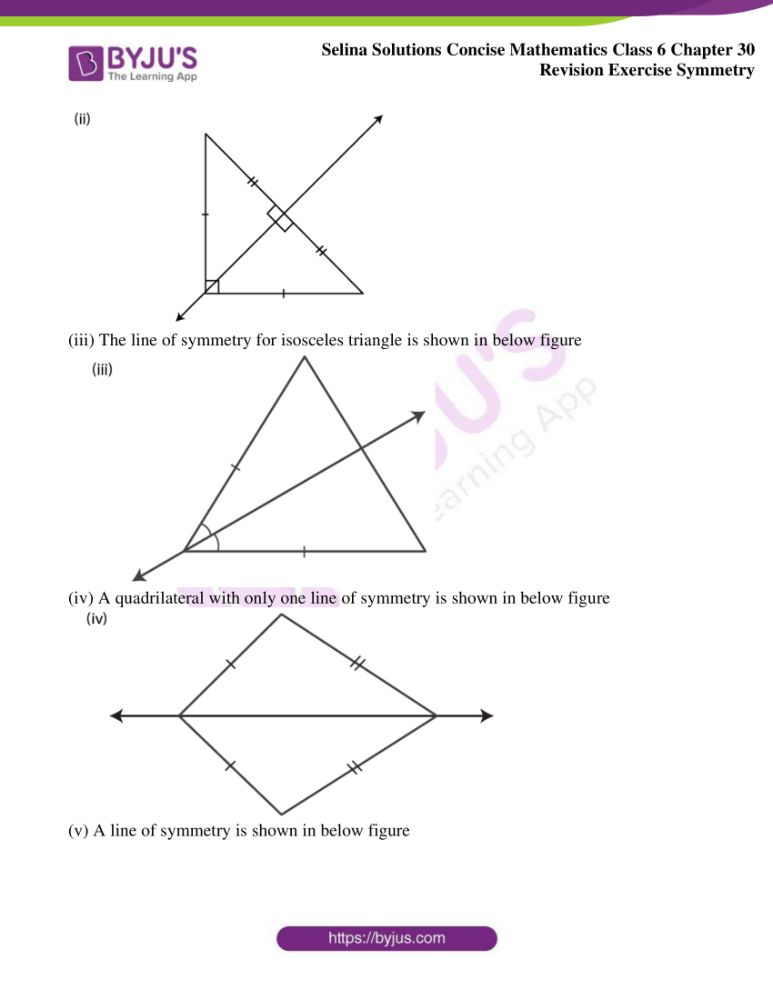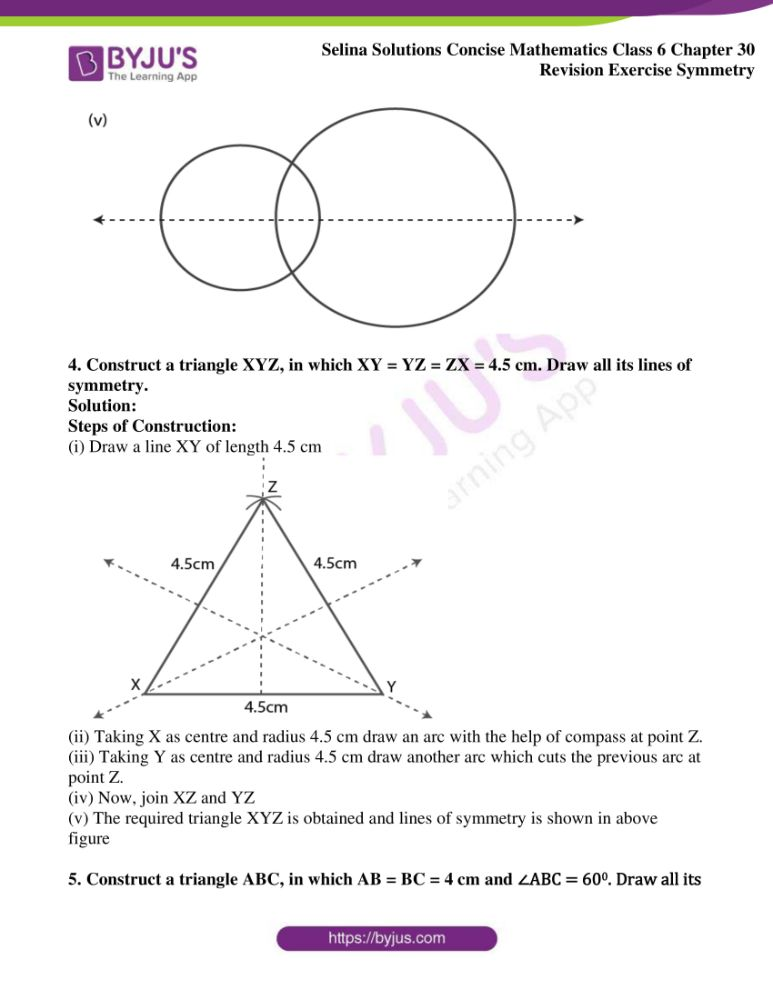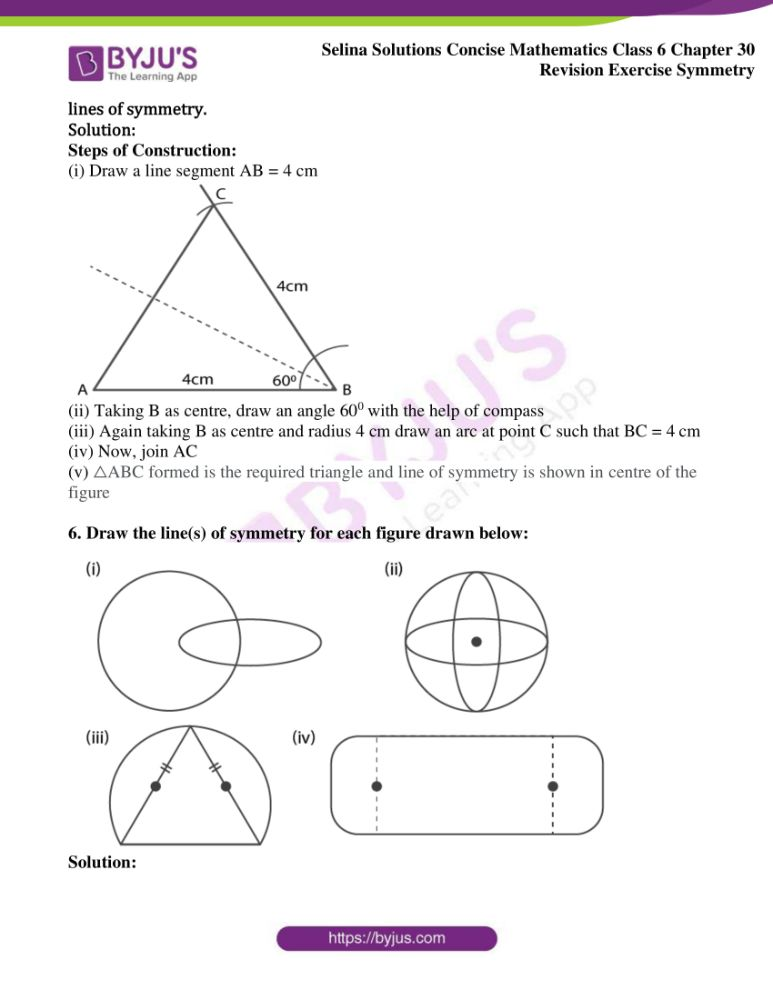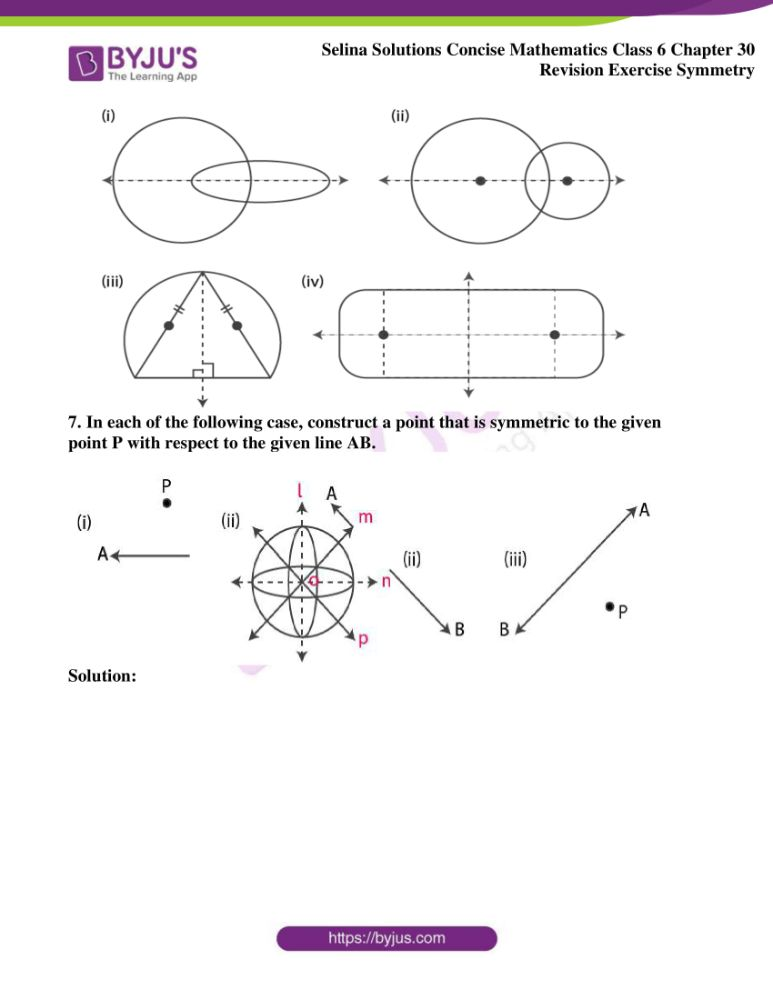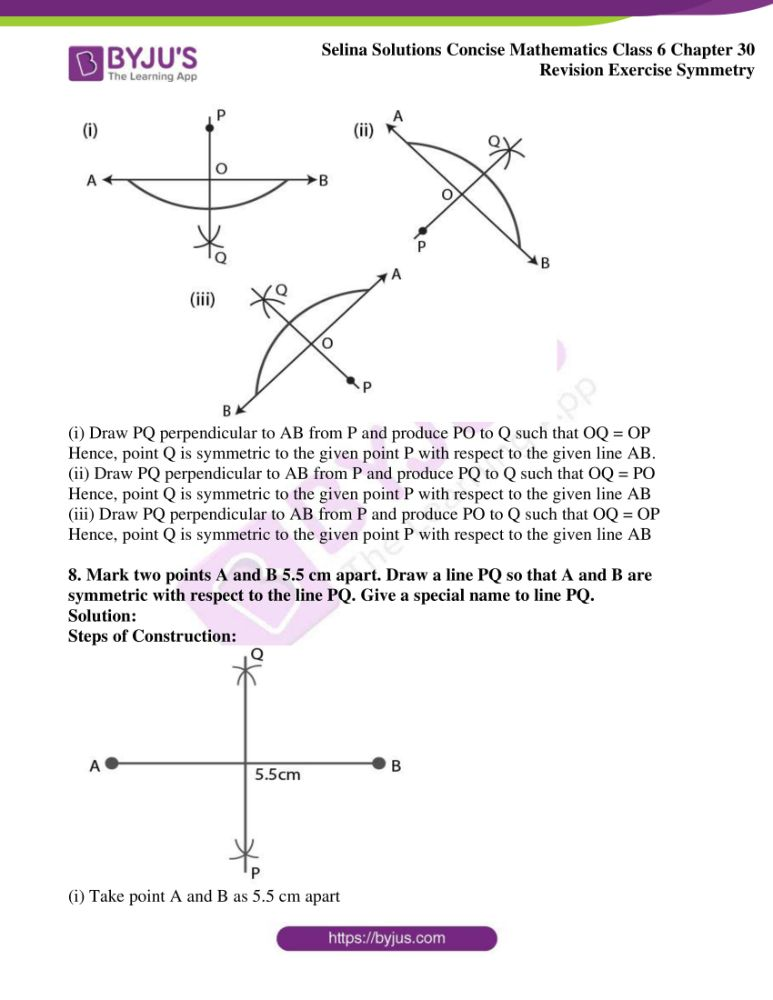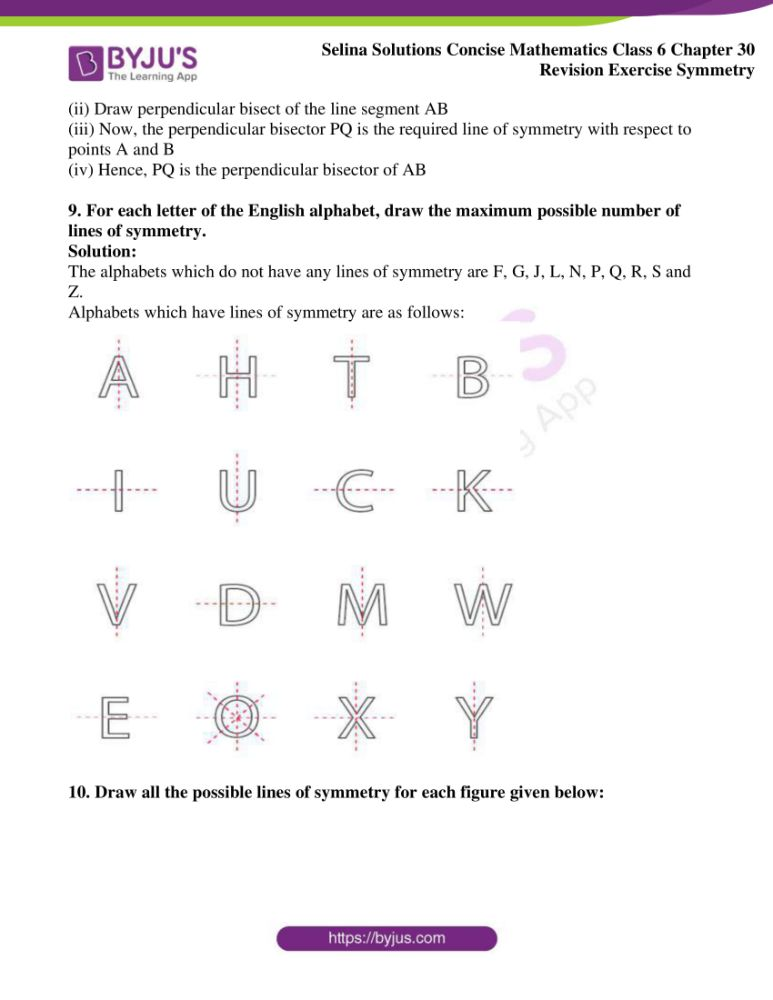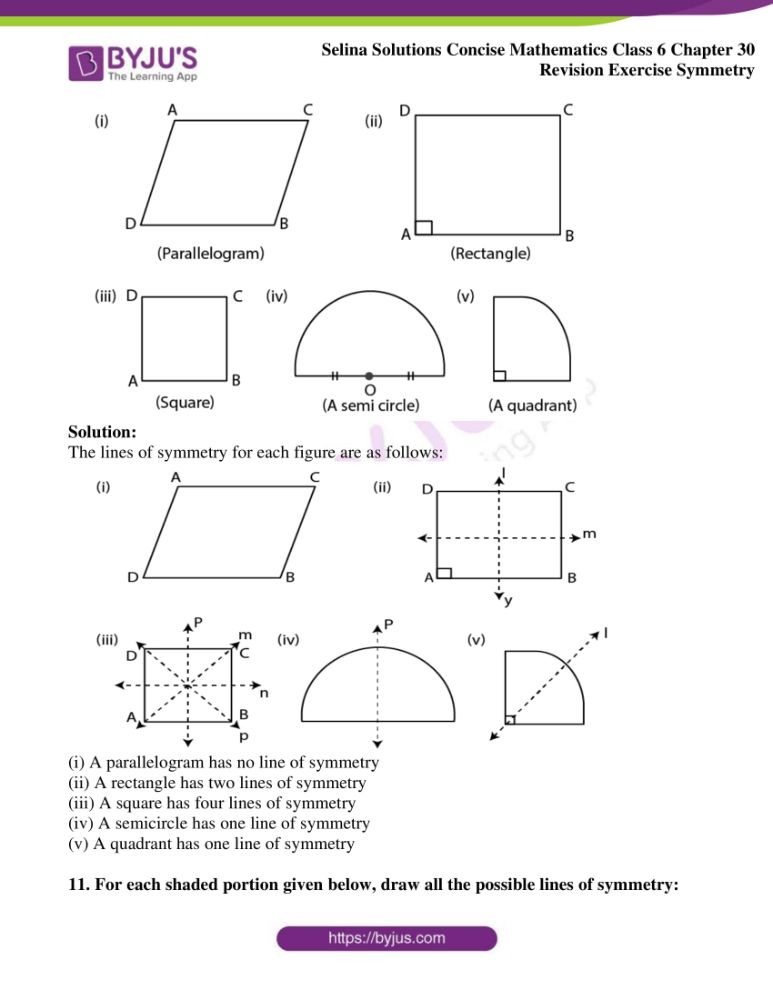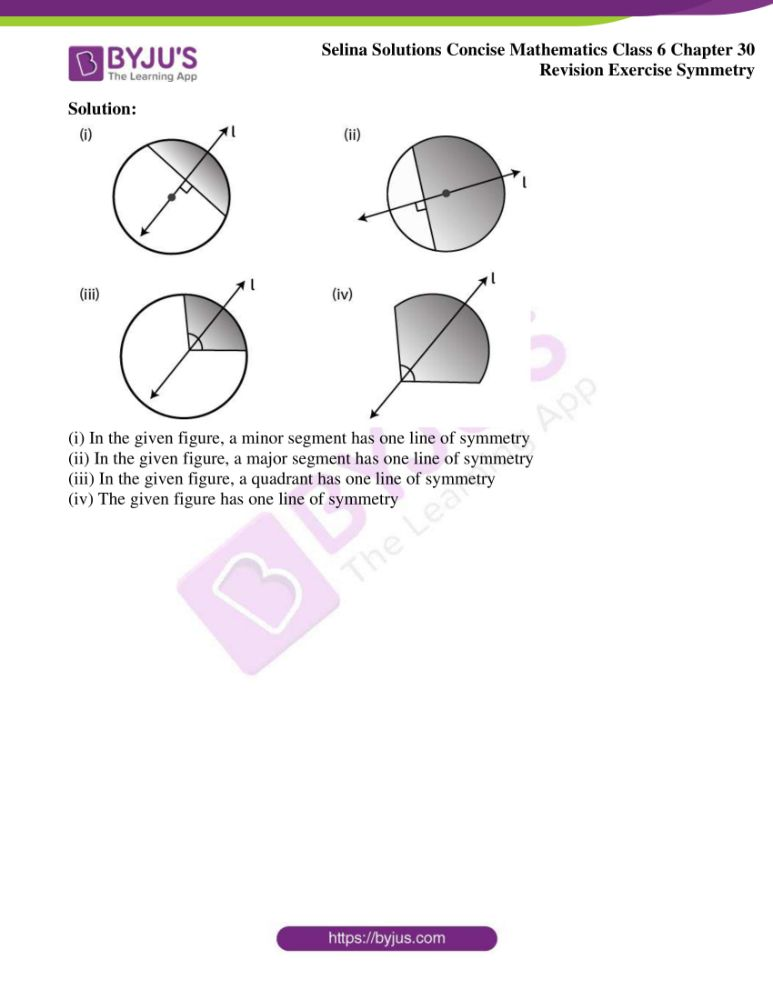### Access Selina Solutions Concise Mathematics Class 6 Chapter 30 Revision Exercise Symmetry

Exercise 30

1. State, whether true or false:

(i) The letter B has one line of symmetry

(ii) The letter F has no line of symmetry

(iii) The letter O has only two lines of symmetry

(iv) The figure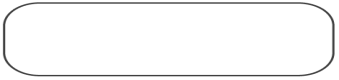has no line of symmetry

(v) The letter N has one line of symmetry

Solution:

(i) The letter B has one line of symmetry. Hence, the given statement is true

(ii) The letter F has no line of symmetry. Hence, the given statement is true

(iii) The letter O has many line of symmetry. Hence, the given statement is false

(iv) The figure has two line of symmetry. Hence, the given statement is false

(v) The letter N has no line of symmetry. Hence, the given statement is false

2. Construct a triangle ABC, in which AB = AC = 5 cm and BC = 6 cm. Draw all its lines of Symmetry.

Solution:

Steps of Construction:

(i) Draw a line BC of length 6 cm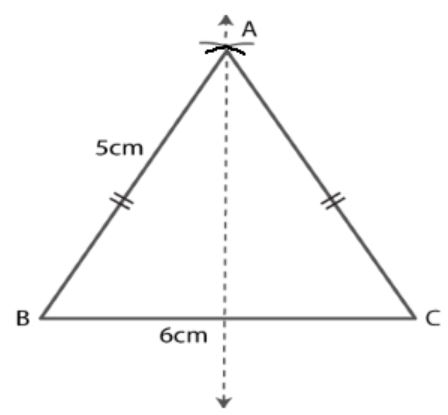(ii) With the help of compass, taking B as centre and radius 5 cm, draw an arc

(iii) Now, taking C as centre and radius 5 cm draw another arc which cuts the previous arc at point A

(iv) Join AB and AC

(v) The required triangle ABC is obtained. Line of symmetry is shown in above figure

3. Examine each of the following figures carefully, draw line(s) of symmetry in which ever figure possible: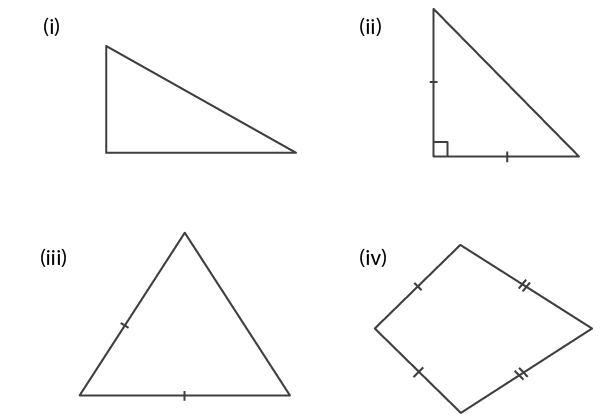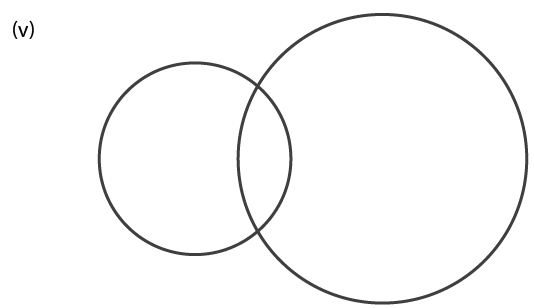Solution:

(i) There is no line of symmetry in the given figure

(ii) The line of symmetry for isosceles right angled triangle is shown in figure below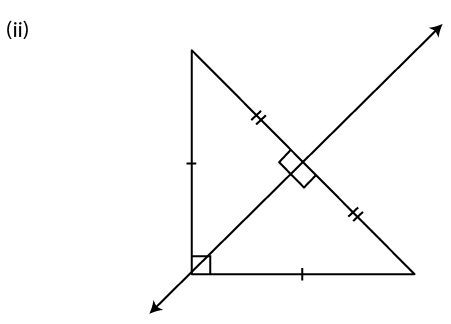(iii) The line of symmetry for isosceles triangle is shown in below figure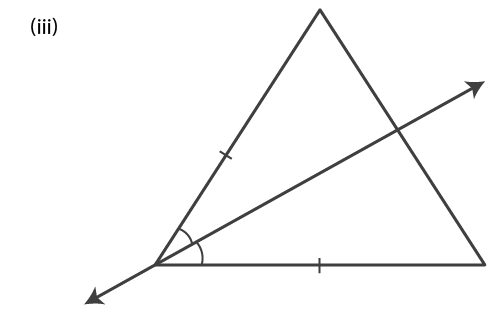(iv) A quadrilateral with only one line of symmetry is shown in below figure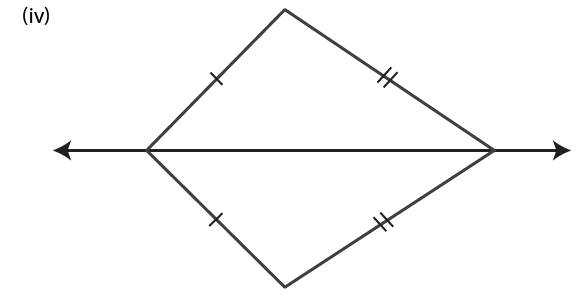(v) A line of symmetry is shown in below figure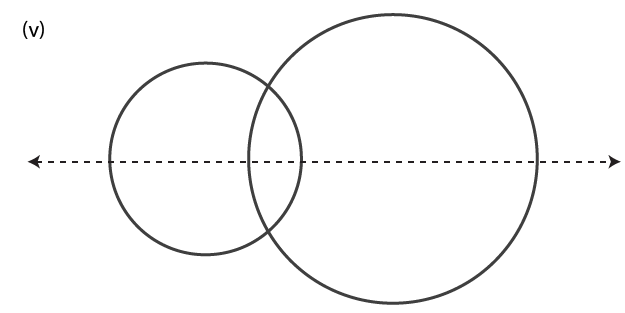4. Construct a triangle XYZ, in which XY = YZ = ZX = 4.5 cm. Draw all its lines of symmetry.

Solution:

Steps of Construction:

(i) Draw a line XY of length 4.5 cm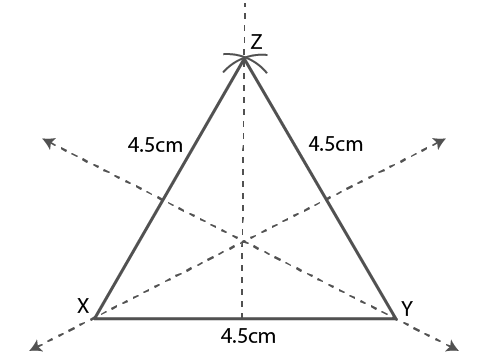(ii) Taking X as centre and radius 4.5 cm draw an arc with the help of compass at point Z.

(iii) Taking Y as centre and radius 4.5 cm draw another arc which cuts the previous arc at point Z.

(iv) Now, join XZ and YZ

(v) The required triangle XYZ is obtained and lines of symmetry is shown in above figure

5. Construct a triangle ABC, in which AB = BC = 4 cm and ∠ABC = 600. Draw all its lines of symmetry.

Solution:

Steps of Construction:

(i) Draw a line segment AB = 4 cm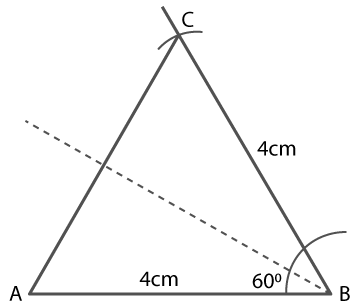(ii) Taking B as centre, draw an angle 600 with the help of compass

(iii) Again taking B as centre and radius 4 cm draw an arc at point C such that BC = 4 cm

(iv) Now, join AC

(v) △ABC formed is the required triangle and line of symmetry is shown in centre of the figure

6. Draw the line(s) of symmetry for each figure drawn below: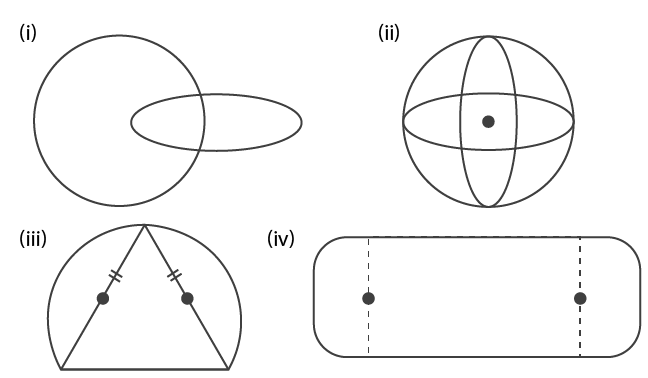Solution: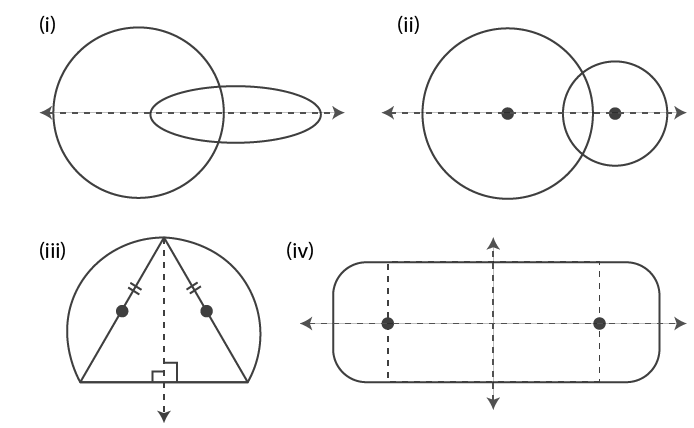7. In each of the following case, construct a point that is symmetric to the given point P with respect to the given line AB.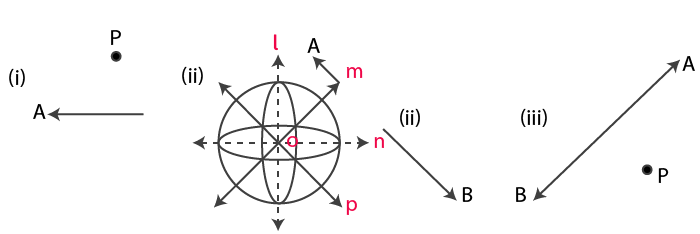Solution: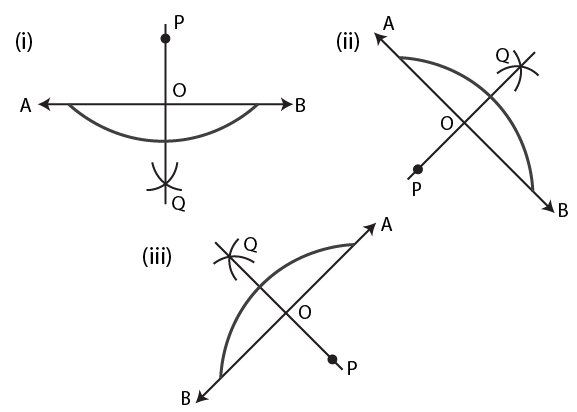(i) Draw PQ perpendicular to AB from P and produce PO to Q such that OQ = OP

Hence, point Q is symmetric to the given point P with respect to the given line AB.

(ii) Draw PQ perpendicular to AB from P and produce PQ to Q such that OQ = PO

Hence, point Q is symmetric to the given point P with respect to the given line AB

(iii) Draw PQ perpendicular to AB from P and produce PO to Q such that OQ = OP

Hence, point Q is symmetric to the given point P with respect to the given line AB

8. Mark two points A and B 5.5 cm apart. Draw a line PQ so that A and B are symmetric with respect to the line PQ. Give a special name to line PQ.

Solution:

Steps of Construction: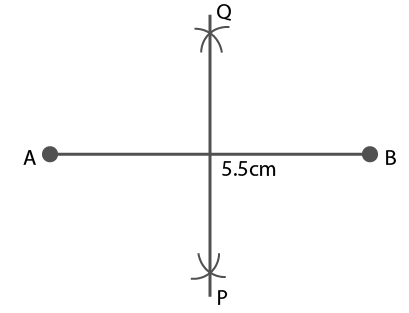(i) Take point A and B as 5.5 cm apart

(ii) Draw perpendicular bisect of the line segment AB

(iii) Now, the perpendicular bisector PQ is the required line of symmetry with respect to points A and B

(iv) Hence, PQ is the perpendicular bisector of AB

9. For each letter of the English alphabet, draw the maximum possible number of lines of symmetry.

Solution:

The alphabets which do not have any lines of symmetry are F, G, J, L, N, P, Q, R, S and Z.

Alphabets which have lines of symmetry are as follows: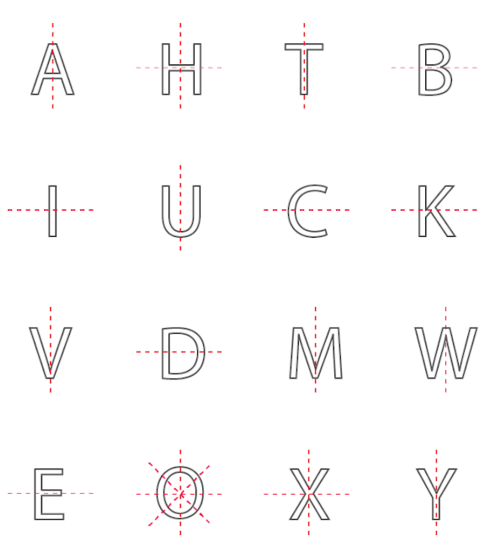10. Draw all the possible lines of symmetry for each figure given below: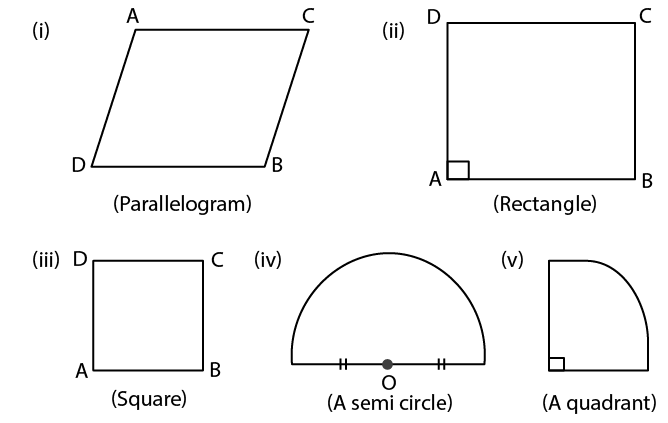Solution:

The lines of symmetry for each figure are as follows: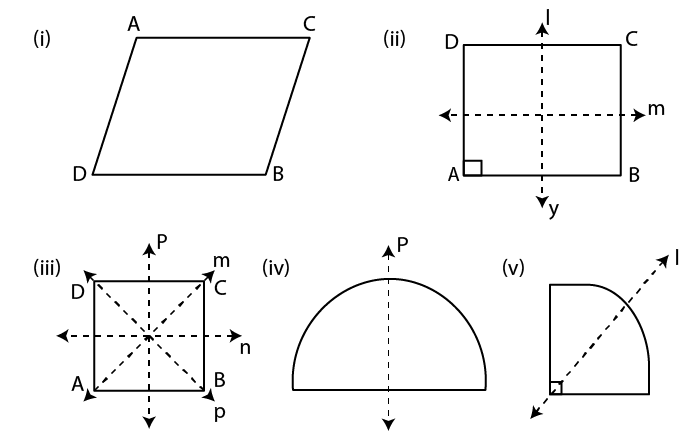(i) A parallelogram has no line of symmetry

(ii) A rectangle has two lines of symmetry

(iii) A square has four lines of symmetry

(iv) A semicircle has one line of symmetry

(v) A quadrant has one line of symmetry

11. For each shaded portion given below, draw all the possible lines of symmetry:

Solution: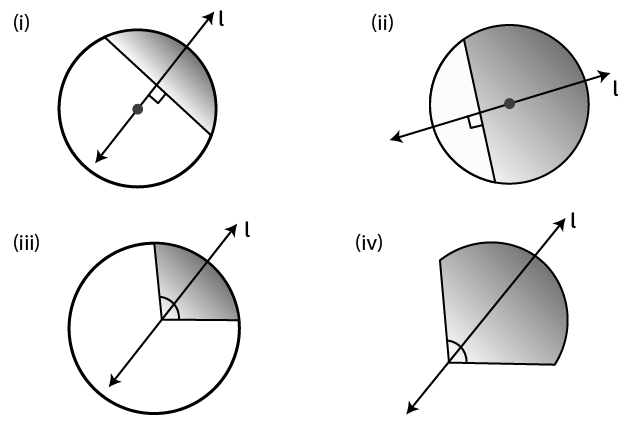(i) In the given figure, a minor segment has one line of symmetry

(ii) In the given figure, a major segment has one line of symmetry

(iii) In the given figure, a quadrant has one line of symmetry

(iv) The given figure has one line of symmetry